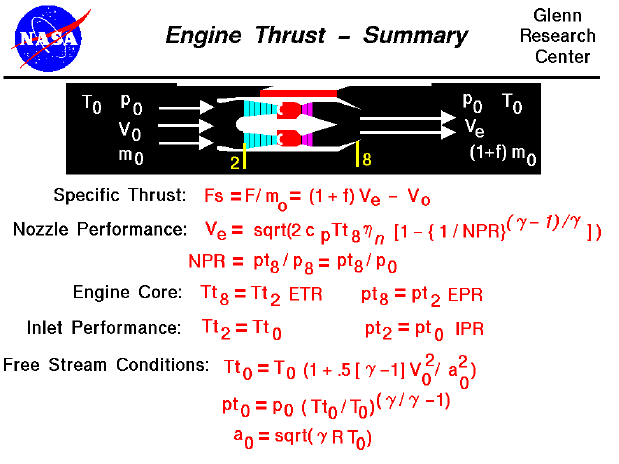A text only version of this slide is available which gives all of the flow equations. The interactive Java applet EngineSim is also available. This program solves these equations and displays the thrust and fuel flow values for a variety of turbine engines.

On this slide we have gathered together all of the equations necessary to compute the theoretical thrust for a turbojet engine. The general thrust equation is given just below the graphic in the specific thrust form. The specific thrust depends only on the exit velocity from the nozzle, the free stream velocity, and the fuel to air ratio, f. The equation for the exit velocity was developed on the nozzle performance slide and depends on some thermodynamic properties, the total temperature in the nozzle, and the nozzle pressure ration (NPR). The NPR is simply the ratio of the total pressure in the nozzle to the free stream static pressure. The total pressure (pt8) and the total temperature (Tt8) in the nozzle depend on the engine pressure ratio (EPR) and temperature ratio (ETR). The equations for these ratios are given on separate slides and depend on the pressure and temperature ratio across each of the engine components. The engine pressure and temperature ratios are referenced to conditions at the compressor face. Compressor face conditions depend on free stream conditions and inlet performance. The equations at the bottom of the slide are compressible forms for determining the total pressure and temperature conditions from the corresponding static conditions and the free stream velocity. The variable "a0" is the free stream speed of sound.Guided Tours